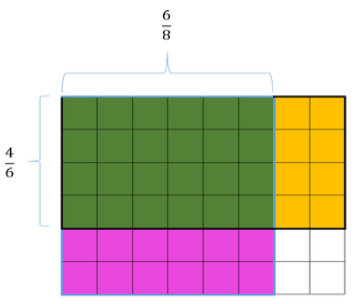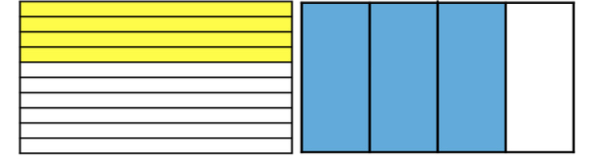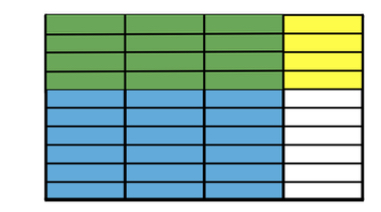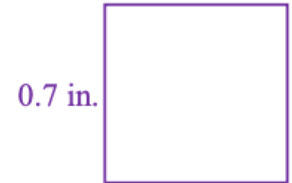# MA.5.GR.2.1Export Print
Find the perimeter and area of a rectangle with fractional or decimal side lengths using visual models and formulas.

### Clarifications

Clarification 1: Instruction includes finding the area of a rectangle with fractional side lengths by tiling it with squares having unit fraction side lengths and showing that the area is the same as would be found by multiplying the side lengths.

Clarification 2: Responses include the appropriate units in word form.

General Information
Subject Area: Mathematics (B.E.S.T.)
Strand: Geometric Reasoning
Status: State Board Approved

## Benchmark Instructional Guide

• Area Model
• Perimeter

### Vertical Alignment

Previous Benchmarks

Next Benchmarks

### Purpose and Instructional Strategies

The purpose of this benchmark is for students to understand how to work with fractional and decimal sums and products when calculating perimeter and area. This benchmark connects to previous work where students found areas and perimeters with whole number side lengths in grade 4 (MA.4.GR.2.1) and prepares for future work of finding area and perimeter on a coordinate plane in grade 6 (MA.6.GR.1.3).
• During instruction, teachers should encourage students to use models or drawings to assist them with finding the perimeter and area of a rectangle and have them explain how they used the model or drawing to arrive at the solution getting them to understand that multiplying fractional side lengths to find the area is the same as tiling a rectangle with unit squares of the appropriate unit fraction side lengths (MTR.5.1).
• This benchmark provides a natural real-world context and also a visual model for the multiplication of fractions and decimals. When finding the area, teachers can begin with students modeling multiplication with whole numbers and progress into the fractional and decimal parts, such as area models using rectangles or squares, fraction strips/bars and sets of counters.
• For example, ask questions such as, “What does 2 × 3 mean?” Then, follow with questions for multiplication with fractions, such as, “What does $\frac{\text{3}}{\text{4}}$× $\frac{\text{1}}{\text{3}}$ mean?” “What does $\frac{\text{3}}{\text{4}}$ × 7 mean?” (7 sets of $\frac{\text{3}}{\text{4}}$) and “What does 7 × $\frac{\text{3}}{\text{4}}$ mean?” ($\frac{\text{3}}{\text{4}}$of a set of 7) (MTR.2.1, MTR.3.1, MTR.5.1).

### Common Misconceptions or Errors

• Students may believe that multiplication always results in a larger number. Working with area provides them with concrete situations where this is not true.
• For example a city block that is $\frac{\text{1}}{\text{10}}$ mile by $\frac{\text{1}}{\text{10}}$ mile has an area of 100 of a square mile.
• Students may have difficulty connecting visual models to the symbolic representation using equations. Use concrete visuals to represent problems.

### Strategies to Support Tiered Instruction

• Instruction provides opportunities to use concrete visuals to represent problems. Instruction includes providing a rectangle to divide into fractional parts. The teacher provides students with fractional dimensions to divide the figure into to find the area of part of the whole figure. Before calculating the area, students explain if the area will be greater or less than one of the dimensions and explain how they know.
• For example, the teacher provides students with a blank rectangle and has students divide into fractional parts as shown below. The teacher uses prompts like those shown to help guide the students. After dividing the figure, the students use two different colors to shade the fractional parts and label each side with the shaded dimensions ($\frac{\text{6}}{\text{8}}$ or $\frac{\text{4}}{\text{6}}$).
• Divide the figure vertically into eights. Divide the figure horizontally into sixths. Shade $\frac{\text{6}}{\text{8}}$ vertically and $\frac{\text{4}}{\text{6}}$ horizontally. The area of$\frac{\text{6}}{\text{8}}$ × $\frac{\text{4}}{\text{6}}$ is where the 2 shaded sections overlap. Is the shaded area greater or less than $\frac{\text{6}}{\text{8}}$? How do you know?• Instruction includes providing fractional area models printed on transparency sheets. Models include equal size wholes divided into thirds, fourths, fifths, sixths, eighths, tenths, and twelfths. Students use two transparencies to show the area of given dimensions.
• For example, the the teacher asks students to find the area of a figure with side lengths of $\frac{\text{3}}{\text{4}}$ inch and $\frac{\text{4}}{\text{10}}$ inch. Students model $\frac{\text{3}}{\text{4}}$ × $\frac{\text{4}}{\text{10}}$ by shading $\frac{\text{3}}{\text{4}}$ of one fraction model and $\frac{\text{4}}{\text{10}}$ of another fraction model. The teacher has students explain if the area will be greater or less than $\frac{\text{3}}{\text{4}}$ and explain how they know. The students then overlap the two figures and determine the fractional parts that overlap as being the area.• Image showing $\frac{\text{4}}{\text{10}}$ overlapping $\frac{\text{3}}{\text{4}}$, $\frac{\text{12}}{\text{40}}$ is overlapping.Margaret draws a rectangle with a length of 5.2 inches. The width of her rectangle is one-half its length.
• Part A. Draw Margaret’s rectangle and show its dimensions.
• Part B. What is the perimeter of her rectangle in inches?
• Part C. What is the area of her rectangle in square inches?

### Instructional Items

Instructional Item 1

What is the area of the square below?*The strategies, tasks and items included in the B1G-M are examples and should not be considered comprehensive.

## Related Courses

This benchmark is part of these courses.
1200400: Foundational Skills in Mathematics 9-12 (Specifically in versions: 2014 - 2015, 2015 - 2022, 2022 and beyond (current))
5012070: Grade Five Mathematics (Specifically in versions: 2014 - 2015, 2015 - 2022, 2022 and beyond (current))
7712060: Access Mathematics Grade 5 (Specifically in versions: 2014 - 2015, 2015 - 2018, 2018 - 2022, 2022 and beyond (current))
5012065: Grade 4 Accelerated Mathematics (Specifically in versions: 2019 - 2022, 2022 and beyond (current))
5012015: Foundational Skills in Mathematics 3-5 (Specifically in versions: 2019 - 2022, 2022 and beyond (current))

## Related Access Points

Alternate version of this benchmark for students with significant cognitive disabilities.
MA.5.GR.2.AP.1: Find the perimeter and area of a rectangle with decimal side lengths using a visual model and calculator.

## Related Resources

Vetted resources educators can use to teach the concepts and skills in this benchmark.

## Formative Assessment

The Rectangle:

Students determine the area of a rectangle with given fractional dimensions by multiplying. Students are then asked to draw a model to find the area of the same rectangle.

Type: Formative Assessment

## Lesson Plan

Bill of Rights Billboard:

This MEA will deepen students' knowledge of the Bill of Rights through collaborative problem solving. Students are required to analyze data in order to recommend three Amendments to celebrate during a community festival.  They will perform operations with fractions and mixed numbers to recommend advertising options for the festival within a budget.

Type: Lesson Plan

## STEM Lessons - Model Eliciting Activity

Bill of Rights Billboard:

This MEA will deepen students' knowledge of the Bill of Rights through collaborative problem solving. Students are required to analyze data in order to recommend three Amendments to celebrate during a community festival.  They will perform operations with fractions and mixed numbers to recommend advertising options for the festival within a budget.

## MFAS Formative Assessments

The Rectangle:

Students determine the area of a rectangle with given fractional dimensions by multiplying. Students are then asked to draw a model to find the area of the same rectangle.

## Student Resources

Vetted resources students can use to learn the concepts and skills in this benchmark.

## Parent Resources

Vetted resources caregivers can use to help students learn the concepts and skills in this benchmark.